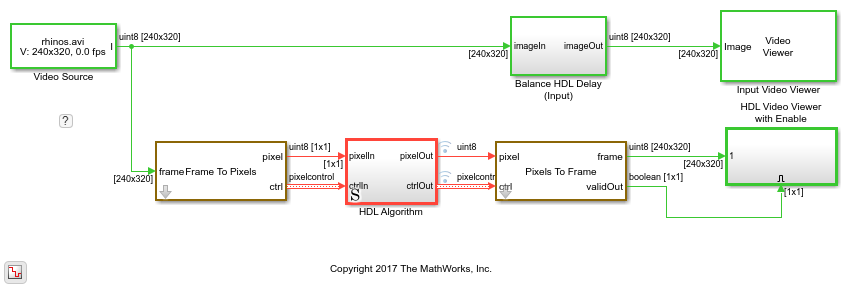# Construct a Filter Using Line Buffer

This example shows how to use the Line Buffer block to extract neighborhoods from an image for further processing. The model constructs a separable Gaussian filter.Inside the HDL Algorithm subsystem, the Line Buffer block is configured for a 5-by-5 neighborhood. The output is a 5-by-1 column vector. The Gain and Sum blocks implement separate horizontal and vertical components of a 5-by-5 Gaussian filter with a 0.75 standard deviation. After vertical filtering, the model stores the column sums in a shift register that creates a 1-by-5 row vector. The row values are filtered again to calculate the new central pixel value of each neighborhood.You can generate HDL code from the HDL Algorithm subsystem. You must have the HDL Coder™ software installed to run this command.

```makehdl('SeparableFilterSimpleHDL/HDL Algorithm') ```

To generate an HDL test bench, use this command.

```makehdltb('SeparableFilterSimpleHDL/HDL Algorithm') ```